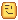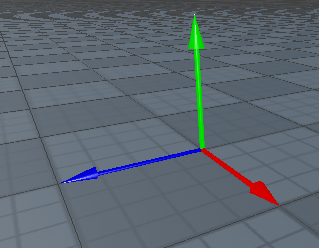# Can anyone explain this code?

I actually stumbled across a code

``````If humanoid.MoveDirection.magnitude <= 50 then
-- condition
end
``````

Well I couldn’t understand how this works please explain.

Well so i see you don’t know about magnitude,physics,Direction,CFrames.

Let me explain them.

Basically that direction is the Direction where the Body/Character of player is Moving to.Note: It’s a Direction Not a Position even though both are Vector3. Now let’s talk about magnitude. Magnitude is the amount of studs a position is far from.
For example the Magnitude of 0,10,0 is 10 studs because it’s ten studs for from the origin.

Now this is checking if the distance in studs is less than or equivalent of the direction the humanoid is moving in and if it is it will execute the code in the next block.

Hope this helps1 Like

To add on what @IamNewToRiblix said, people generally use `if humanoid.MoveDirection.Magnitude <= X then` to check if the humanoid is moving or not.

For example, you could use `humanoid.MoveDirection.Magnitude <= 0 then` to check if the player is idle and not moving.

Humanoid.MoveDirection returns a vector3, which has 3 values:Green = Y.
Blue = Z.
Red = X.

The humanoid has a function called :MoveTo(Vector3.new(X,Y,Z)), which walks the character to a certain point. :MoveTo() sets the MoveDirection to the given vector3 in :MoveTo.

Now, if we gather all those values and create a length we can detect if the humanoid is moving by doing that code listed above. Though it’s usually done like this:

``````if humanoid.MoveDirection.magnitude > 0 then
-- walking
else
-- not walking
end
``````

Hopefully this helps. But I literally paid zero attention to math in school so some of this is probably wrong

1 Like

Yea i forgor that. Thx @Downrest and @no_tsi

1 Like

Thnx Devs well I’m about CFrame and stuffs but I didn’t know about move direction moveto blah blah blah …